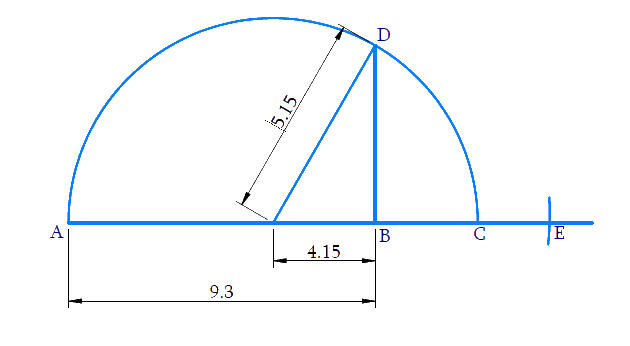# Exercise 1.5 Number Systems NCERT Solutions Class 9

Go back to  'Number Systems'

## Chapter 1 Ex.1.5 Question 1

Classify the following numbers as rational or irrational:

(i) \begin{align} \quad 2 - \sqrt 5 \end{align}

(ii) \begin{align}(3 + \sqrt {23} ) - \sqrt {23} \end{align}

(iii) \begin{align}\frac{{2\sqrt 7 }}{{7\sqrt 7 }} \end{align}

(iv) \begin{align}\frac{1}{{\sqrt 2 }} \end{align}

(v) \begin{align}2\pi \end{align}

### Solution

\begin{align}({\rm{i}})\,\,\,\,2 - \sqrt 5 \end{align}

Steps:

The sum or difference of a rational number and an irrational number is always irrational.

Here $$2$$ is a rational number and \begin{align} \sqrt{5} \end{align} is an irrational number. Hence \begin{align} 2 -\sqrt{5} \end{align} is an irrational number.

\begin{align}\rm{(ii)}\quad(3 + \sqrt {23} ) - \sqrt {23} \end{align}

Steps:

By simplifying we get only $$3$$.

\begin{align}3 = \frac{3}{1},\end{align} which is in the form of \begin{align}\frac{p}{q},\end{align}

Hence is \begin{align}(3 + \sqrt {23} ) - \sqrt {23} \end{align} a rational number.

\begin{align}\rm{(iii)}\quad \frac{{2\sqrt 7 }}{{7\sqrt 7 }}\end{align}

Steps:

\begin{align}\frac{{2\sqrt 7 }}{{7\sqrt 7 }} = \frac{2}{7},\end{align} which is in the form of \begin{align}\frac{p}{q},\end{align}

Hence \begin{align}\frac{{2\sqrt 7 }}{{7\sqrt 7 }}\end{align} is a rational number.

\begin{align}\rm{(iv)}\quad \frac{1}{{\sqrt 2 }}\end{align}

Steps:

\begin{align} \frac{1}{\sqrt{2}} &=\frac{1}{\sqrt{2}} \times \frac{\sqrt{2}}{\sqrt{2}} \\ &=\frac{\sqrt{2}}{2} \\ &=\frac{1.414 \ldots}{2} \end{align}

\begin{align}= 0.702……\end{align} is a non - terminating, non-recurring decimal  and therefore it is irrational. Hence \begin{align}\,\frac{1}{{\sqrt 2 }}\,\end{align} is an irrational number.

\begin{align}\rm{(v)}\quad 2{\rm{\pi }}\end{align}

Steps:

\begin{align}~2\text{ }\!\!\pi\!\!\text{ }=2\times 3.1415...\end{align}

$$\pi$$ is an irrational number whose value is non-terminating and non-recurring.

$$2$$ is a rational number.

Product of a non-zero rational number and irrational number is an irrational number.

Hence \begin{align}2\pi\end{align} is irrational.

## Chapter 1 Ex.1.5 Question 2

Simplify each of the following expressions

(i) $$(3 + \sqrt 3 )\,(2 + \sqrt 2 )$$

(ii) $$(3 + \sqrt 3 )\,(3 - \sqrt 3 )$$

(iii)$${(\sqrt 5 + \sqrt 2 )^2}$$

(iv) $$(5 - \sqrt 2 )\,(5 + \sqrt 2 )$$

### Solution

Steps:

(i) \begin{align}(3 + \sqrt 3 )\,(2 + \sqrt 2 )\end{align}

By Distributive property

\begin{align}=6+3 \sqrt{2}+2 \sqrt{3}+\sqrt{6} \end{align}

(ii) \begin{align}(3 + \sqrt 3 )\,(3 - \sqrt 3 )\end{align}

Using the identity:

\begin{align}(a+b)(a-b) &=a^{2}-b^{2} \3+\sqrt{3}) 3-\sqrt{3} &=9-3 \\ &=6 \end{align} (iii) \(\begin{align}{(\sqrt 5 + \sqrt 2 )^2}\,\end{align}

Steps:

Using the identity: $$(a+b)^{2} =a^{2}+2 a b+b^{2}$$

\begin{align}&=(\sqrt{5})^{2}+2 \times \sqrt{5} \times \sqrt{2}+(\sqrt{2})^{2} \\ &=(5+2 \sqrt{10}+2) \\ &=7+2 \sqrt{10} \end{align}

(iv) \begin{align}(5 - \sqrt 2 )\,(5 + \sqrt 2 )\end{align}

Steps:

Using the identity: $$(a+b)(a-b) =a^{2}-b^{2}$$

\begin{align}&(\sqrt{5})^{2}-(\sqrt{2})^{2} \\ &=5-2 \\ &=3 \end{align}

## Chapter 1 Ex.1.5 Question 3

Recall, \begin{align}\pi \end{align} is defined as the ratio of circumference (say $$c$$) to its diameter (say $$d$$). That is \begin{align}{\rm{\pi = }}\frac{c}{d}.\end{align}. This seems to contradict the fact that \begin{align}\pi \end{align} is irrational. How will you resolve this contradiction?

### Solution

Steps:

Writing $$\pi$$ as \begin{align}\frac{22}{7}\end{align} is only an approximate value and so we can’t conclude that it is in the form of a rational. In fact, the value of $$\pi$$ is calculating as non-terminating, non-recurring decimal as $$\pi=3.14159\dots$$ Whereas

If we calculate the value of \begin{align}\frac{22}{7}\end{align} it gives $$3.142857$$ and hence\begin{align}\pi\ne\frac{22}{7}\end{align}

In conclusion $$\pi$$ is an irrational number.

## Chapter 1 Ex.1.5 Question 4

Represent \begin{align}\sqrt {9.3} \end{align} on the number line.

### Solution

Steps:

Draw a line and take $$AB = 9.3$$ units on it.

From $$B$$ measure a distance of $$1$$ unit and mark $$C$$ on the number line. Make the midpoint of $$AC$$ as $$O.$$

With $$‘O’$$ as center and $$OC$$ as radius, draw a semicircle.

At $$B$$, draw a perpendicular to cut the semicircle at $$D$$, with $$B$$ as center and $$BD$$ as radius draw an arc to cut the number line at $$E$$. Taking $$B$$ as the origin the distance $${BE}=\sqrt{9.3}$$ and hence $$E$$ represents $$\sqrt{9.3}$$ .Proof:

\begin{align}AB &=9.3, BC=1 \\ AC &=A B+BC=10.3 \\ OC &=\frac{AC}{2}=\frac{10.3}{2}=5.15 \\ OC&=OD=5.15\\O B &=OC-BC\\&=5.15-1=4.15 \end{align}

In right angled\begin{align}\Delta OBD,\end{align}

\begin{align} {BD}^{2} &={OD}^{2}-{OB}^{2}\\ &=(5.15)^{2}-(4.15)^{2} \\ &=(5.15+4.15)(5.15-4.15)\\&\rm{Using} \quad a^{2}-b^{2}=(a+b)(a-b) \\ &=9.3 \times 1\\ &=9.3 \\ BD &=\sqrt{9.3}=BE \end{align}

## Chapter 1 Ex.1.5 Question 5

Rationalize the denominators of the following:

(i) \begin{align}\frac{1}{{\sqrt 7 }} \end{align}

(ii)\begin{align}\frac{1}{{\sqrt 7 - \sqrt 6 }}\end{align}

(iii) \begin{align}\frac{1}{{\sqrt 5 + \sqrt 2 }}\end{align}

(iv) \begin{align}\frac{1}{{\sqrt 7 - 2}}\end{align}

### Solution

Steps:

(i) \begin{align}\frac{1}{{\sqrt 7 }} = \frac{1}{{\sqrt 7 }} \times \frac{{\sqrt 7 }}{{\sqrt 7 }}\end{align}

(Dividing and multiplying by $$\sqrt{7}$$ )

$=\frac{\sqrt{7}}{7}$

(ii) \begin{align}\frac{1}{{\sqrt 7 - \sqrt 6 }}\end{align}

Steps:

Dividing and multiplying by \begin{align}\sqrt 7 + \sqrt 6 \end{align}, we get

\begin{align} &=\frac{1}{\sqrt{7}-\sqrt{6}} \times \frac{\sqrt{7}+\sqrt{6}}{\sqrt{7}-\sqrt{6}} \\ &=\frac{\sqrt{7}+\sqrt{6}}{(\sqrt{7})^{2}-(\sqrt{6})^{2}}\\&\left(\text { Using }(a+b)(a-b)=a^{2}-b^{2}\right) \\ &=\frac{\sqrt{7}+\sqrt{6}}{7-6} \\ &=\sqrt{7}+\sqrt{6} \end{align}

(iii) \begin{align}\frac{1}{{\sqrt 5 + \sqrt 2 }}\end{align}

Steps:

Dividing and multiplying by \begin{align}\sqrt 5 - \sqrt 2 \end{align} , we get

\begin{align} &=\frac{1}{\sqrt{5}+\sqrt{2}} \times \frac{\sqrt{5}-\sqrt{2}}{\sqrt{5}-\sqrt{2}} \\ &=\frac{\sqrt{5}-\sqrt{2}}{(\sqrt{5})^{2}-(\sqrt{2})^{2}} \\&\text{Using }(a+b)(a-b)=\left(a^{2}-b^{2}\right)\\ &=\frac{\sqrt{5}-\sqrt{2}}{5-2} \\ &=\frac{\sqrt{5}-\sqrt{2}}{3} \end{align}

(iv) \begin{align}\frac{1}{{\sqrt 7 - 2}} & \end{align}

Steps:

Dividing and multiplying by \begin{align}\sqrt 7 + 2,\end{align}, we get

\begin{align} &=\frac{1}{\sqrt{7}-2} \times \frac{\sqrt{7}+2}{\sqrt{7}+2} \\ &=\frac{\sqrt{7}+2}{(\sqrt{7})^{2}-(2)^{2}}\\&\left(\text{Using }(a+b)(a-b)={a}^{2}-{b}^{2}\right)\\ &=\frac{\sqrt{7}+2}{7-4} \\ &=\frac{\sqrt{7}+2}{3} \end{align}

Related Sections
Related Sections
Instant doubt clearing with Cuemath Advanced Math Program